#### 期刊菜单

The Carbon Emission Right Option Pricing and Empirical Analysis under COVID-19
DOI: 10.12677/AAM.2022.115274, PDF, HTML, XML, 下载: 35  浏览: 91  国家自然科学基金支持

Abstract: With the advent of the era of low-carbon economy, the pricing of carbon emission rights has at-tracted more and more attention from both theoretical research and practical application, espe-cially the pricing of carbon emission rights options under the COVID-19. In this paper, with the help of the gradient lifting algorithm of decision tree (LGBM), complete empirical mode decomposition with adaptive noise (CEEMDAN) and the approximate entropy reconstruction (AE) algorithm, and the advantages of the jump-diffusion model of mechanism conversion (RSJM) and the radial basis function neural network model (RBF), a RSJM-RBF carbon emission option pricing model with dif-ferent feature dimensions is established. The empirical analysis is made by using the data of EU-EUA carbon emission option under the COVID-19. The empirical results show that compared with the fractional Brownian motion model (FBM) based on GARCH, the RSJM-RBF carbon emission option pricing model proposed in this paper has higher prediction accuracy. Taking Pearson corre-lation coefficient as the index, the robustness analysis of the two models shows that the model es-tablished in this paper is more robust.

1. 引言

2019年，新冠疫情的突然爆发对全球经济造成了巨大冲击，碳交易市场产生了剧烈的震荡。在全球疫情爆发之初，碳交易市场严重受挫 。欧盟碳排放交易市场作为国际上发展最为成熟的碳交易市场之一，其碳排放权期权价格在2020年3月中旬低至15欧元，虽然在以欧盟为代表的国家推出的绿色发展疫情复苏计划的刺激下   ，碳排放权期权价格有所回升，但此前造成的影响也不容忽视。因此，研究碳排放权期权定价机制及演化规律十分必要。

2. 模型的建立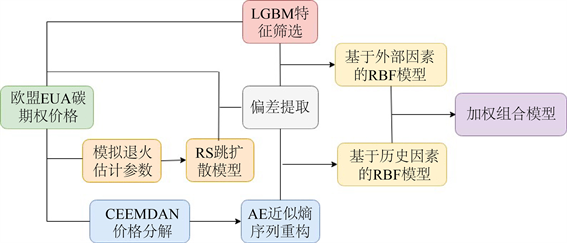Figure 1. Flow chart of model

2.1. LGBM特征工程

2.2. 机制转换的跳–扩散模型(RSJM)

RSJM下的碳排放权期权价格公式为 ：

$V={\int }_{0}^{T}{V}_{0}\left({S}_{0},K,T,\Gamma \right){f}_{{T}_{0}}\left(t|s\left(0\right)=0\right)\text{d}t$ (1)

${f}_{{T}_{0}}\left(t|s\left(0\right)=0\right)$ 为正常经济状态下累积逗留时间的条件概率密度。

${V}_{0}\left({S}_{0},K,T,\Gamma \right)=\underset{{n}_{0}=0}{\overset{\infty }{\sum }}\underset{{n}_{1}=0}{\overset{\infty }{\sum }}\frac{{\text{e}}^{-\left(k+1\right)\left[{\lambda }_{0}t+{\lambda }_{1}\left(T-t\right)\right]}{\left({\lambda }_{0}t\right)}^{{n}_{0}}{\left({\lambda }_{1}\left(T-t\right)\right)}^{{n}_{1}}{\left(k+1\right)}^{n}}{{n}_{0}!{n}_{1}!}V\left({S}_{0},K,T,{r}_{n}^{\ast },{\sigma }_{n}^{\ast }\right)$ (2)

$V\left({S}_{0},K,T,{r}_{n}^{\ast },{\sigma }_{n}^{\ast }\right)={S}_{0}\varphi \left({d}_{n1}^{\ast }\right)-K{\text{e}}^{-rT}\varphi \left({d}_{n2}^{\ast }\right)$ (3)

${\left({\sigma }_{n}^{\ast }\right)}^{2}={\sigma }_{0}^{2}t+{\sigma }_{1}^{2}\left(T-t\right)+\frac{n}{T}{\delta }^{2}$ (4)

${r}_{n}^{\ast }={r}_{0}t+{r}_{1}\left(T-t\right)-{\lambda }_{0}kt-{\lambda }_{1}k\left(T-t\right)+\frac{n}{T}\mathrm{ln}\left(1+k\right)$ (5)

t和 $T-t$ 为正常经济状态( $l=0$)和重大事件发生时经济状态( $l=1$)的累积逗留时间， ${n}_{0}$${n}_{1}$ 分别为两种状态下跳跃次数，总跳跃次数为 $n={n}_{0}+{n}_{1}$Table 1. List of features to be selected

2.3. RBF神经网络模型

$\left\{\begin{array}{l}\mathrm{min}\underset{i=1}{\overset{n}{\sum }}{\left({Y}_{i}-\underset{i=1}{\overset{n}{\sum }}{\omega }_{i}{Z}_{it}\right)}^{2}=11.5\\ \underset{i=1}{\overset{n}{\sum }}{\omega }_{i}=1\end{array}$ (6)

${Z}_{i}=\underset{i=1}{\overset{n}{\sum }}{\omega }_{i}{Z}_{it}$ (7)

3. 实证分析

3.1. 数据来源Table 2. Partial experimental data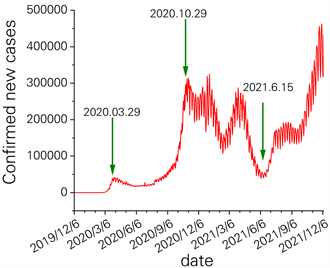Figure 2. Number of daily confirmed cases in EU

3.2. 特征筛选Table 3. Parameter settings of LGBM regression algorithm in different periods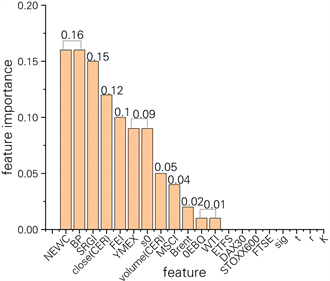Figure 3. Importance of characteristics of epidemic formation period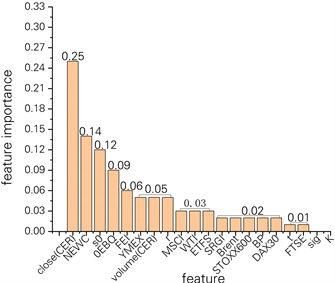Figure 4. Importance of characteristics of an outbreak periodFigure 5. Importance of epidemic fluctuation period characteristics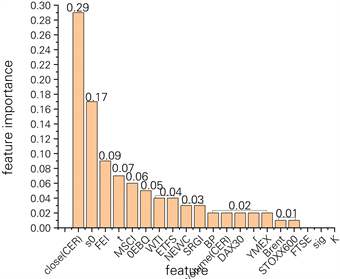Figure 6. Importance of characteristics of secondary eruption period

3.3. 数据分解与重构Table 4. Statistical indicators of each componentTable 5. Statistical indicators of each reconstructed sequence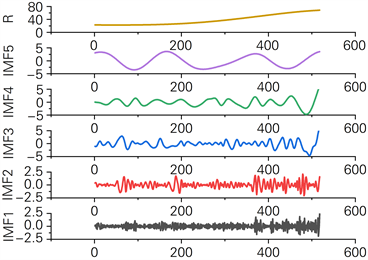Figure 7. EU EUA price breakdown sequence diagram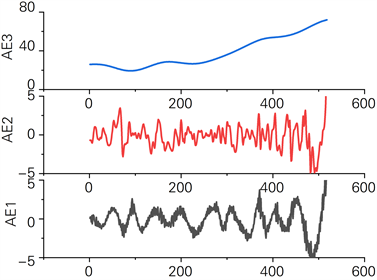Figure 8. Approximate entropy reconstruction sequence of each subsequence

3.4. 欧盟碳排放权期权价格实证分析Table 6. Important parameters of each model

$\left\{\begin{array}{l}\text{MAPE}=\frac{1}{n}\underset{i=1}{\overset{n}{\sum }}|\frac{{y}_{i}-{\stackrel{^}{y}}_{i}}{{y}_{i}}|×100%\\ {\text{R}}^{2}=1-\frac{\underset{i=1}{\overset{n}{\sum }}{\left({y}_{i}-{\stackrel{^}{y}}_{i}\right)}^{2}}{\underset{i=1}{\overset{n}{\sum }}{\left({y}_{i}-{\stackrel{¯}{y}}_{i}\right)}^{2}}\\ \text{MSE}=\frac{1}{n}\underset{i=1}{\overset{n}{\sum }}{\left({y}_{i}-{\stackrel{^}{y}}_{i}\right)}^{2}\end{array}$ (8)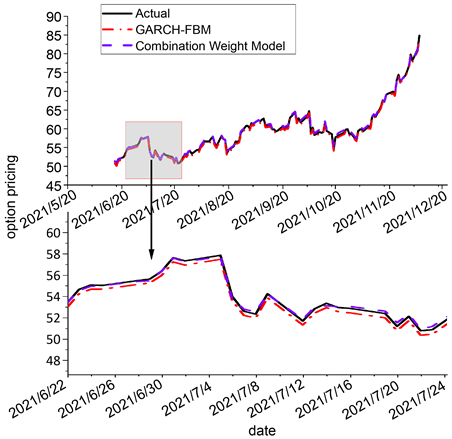Figure 9. Comparative results of two modelsTable 7. Performance comparison of different prediction models of EU carbon option price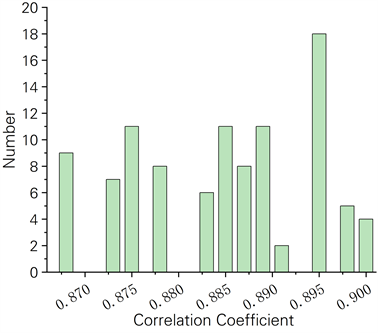Figure 10. Robustness analysis of GARCH-FBM model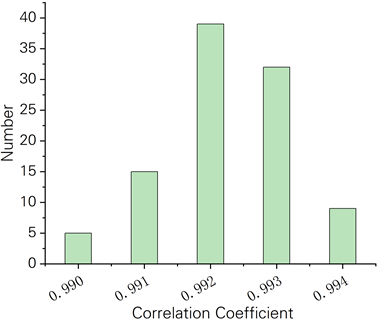Figure 11. Robustness analysis of weight combination model

4. 结论

(一) 针对疫情下碳排放权期权数据的特性，借助于LGBM特征筛选和AE序列重构算法，建立了不同特征维度下RSJM-RBF神经网络模型。

(二) 本文所建模型能够快速响应突发事件下的碳排放权期权价格变化情况，预测精度和鲁棒性均优于参照模型，且比参照模型更能准确预警到期权价格的波动位置和波动幅度。

(三) 根据LGBM特征筛选，同类替代产品中CER结算价和电力价格在不同时期的重要性并未受到较大影响，且一直处于重要地位。宏观经济在各个时期重要性持续处于较低位置，一次能源价格在疫情前重要性显著，疫情爆发之时，重要性整体有所降低。B-S特征中的开盘价在疫情前影响不大，疫情后影响显著。

  田永, 李瑞强, 刘文静. COVID-19疫情前后欧盟碳金融市场的配额拍卖价格及启示——基于碳达峰、碳中和背景[J]. 价格月刊, 2021(8): 1-9.  杨成玉. 欧盟绿色复苏对中欧经贸关系的影响[J]. 国际贸易, 2020(9): 54-60.  王信, 杨娉, 姜晶晶, 等. 疫情冲击对绿色发展和气候相关风险防范的影响[J]. 中国改革, 2020(6): 55-60.  杨成玉. 欧盟复苏计划及其潜在效应[J]. 现代国际关系, 2020(8): 25-32.  江瑞. 基于蒙特卡罗模拟法的中国核证自愿减排量(CCER)碳排放权价值评估研究[D]: [硕士学位论文]. 重庆: 重庆理工大学, 2018.  Liu, Z.B. and Huang, S. (2019) Research on Pricing of Carbon Options Based on GARCH and B-S Model. Journal of Applied Science and Engineering Innovation, 6, 109-116.  韩丹丹. 基于实物期权的企业碳交易决策研究[D]: [硕士学位论文]. 天津: 天津工业大学, 2019.  朱跃钊, 陈红喜, 赵智敏. 基于B-S定价模型的碳排放权交易定价研究[J]. 科技进步与对策, 2013, 30(5): 27-30.  Merton, R.C. (1976) Option Pricing When Un-derlying Stock Returns Are Discontinuous. Journal of Financial Economics, 3, 125-144. https://doi.org/10.1016/0304-405X(76)90022-2  Kou, S.G. (2002) A Jump-Diffusion Model for Option Pric-ing. Management Science, 48, 1086-1101. https://doi.org/10.1287/mnsc.48.8.1086.166  Palao, F. and Pardo, A. (2012) Assessing Price Clustering in Eu-ropean Carbon Markets. Applied Energy, 92, 51-56. https://doi.org/10.1016/j.apenergy.2011.10.022  陈晓红, 王陟昀. 欧洲碳排放权交易价格机制的实证研究[J]. 科技进步与对策, 2010, 27(19): 142-147.  王恺, 邹乐乐, 魏一鸣. 欧盟碳市场期货价格分布特征分析[J]. 数学的实践与认识, 2010, 40(12): 59-65.  张帅, 梁龙跃. 基于Heston模型的上证50ETF期权定价及动态对冲研究[J]. 中国物价, 2021(12): 56-58.  韦伟, 潘泽瀚. 基于Garch模型碳排放期权定价方法研究[J]. 中国外资, 2014(1): 131-132.  徐静, 储盼, 任庆忠. 碳排放权期权定价及实证研究[J]. 统计与决策, 2015(6): 162-165.  Hung, J.C., Liu, H.C. and Yang, J.J. (2020) Improving the Realized GARCH’s Volatility Forecast for Bitcoin with Jump-Robust Estimators. The North American Journal of Economics and Finance, 52, Article ID: 101165. https://doi.org/10.1016/j.najef.2020.101165  张晨, 彭婷, 刘宇佳. 基于GARCH-分形布朗运动模型的碳排放权期权定价研究[J]. 合肥工业大学学报(自然科学版), 2015(38): 1553-1558.  Liu, Z. and Huang, S. (2020) Carbon Option Price Forecasting Based on Modified Fractional Brownian Motion Optimized by GARCH Model in Carbon Emission Trading. The North American Journal of Economics and Finance, 55, Article ID: 101307. https://doi.org/10.1016/j.najef.2020.101307  刘悦, 杨爱军, 林金官. 基于机制转换模型的碳排放权期权定价[J]. 数理统计与管理, 2019, 38(2): 225-234.  宋晓迪. 基于实物期权法的碳资产评估研究[D]: [硕士学位论文]. 合肥: 合肥工业大学, 2017.  李响楚涵. 基于RBF神经网络的实物期权定价研究[D]: [硕士学位论文]. 哈尔滨: 哈尔滨工业大学, 2020.  Li, C.Y., Chen, S.N. and Lin, S.K. (2016) Pricing Derivatives with Modeling CO2 Emission Allowance Using a Regime-Switching Jump Diffusion Model: With Regime-Switching Risk Premium. European Journal of Finance, 22, 887-908. https://doi.org/10.1080/1351847X.2015.1050526  Chevallier, J. (2009) Carbon Futures and Macroeconomic Risk Factors: A View from the EU ETS. Energy Economics, 31, 614-625. https://doi.org/10.1016/j.eneco.2009.02.008  Xu, Y., Luo, M., Tao, L., et al. (2017) ECG Signal De-Noising and Baseline Wander Correction Based on CEEMDAN and Wavelet Threshold. Sensors, 17, 2754. https://doi.org/10.3390/s17122754  Jwa, B., Xin, S.A., Qian, C.A., et al. (2021) An Innovative Random For-est-Based Nonlinear Ensemble Paradigm of Improved Feature Extraction and Deep Learning for Carbon Price Forecast-ing. Science of the Total Environment, 762, Article ID: 143099. https://doi.org/10.1016/j.scitotenv.2020.143099  Zhou, J.G. and Chen, D.F. (2021) Carbon Price Forecasting Based on Improved CEEMDAN and Extreme Learning Machine Optimized by Sparrow Search Algorithm. Sustainability, 13, 4896. https://doi.org/10.3390/su13094896  陈金龙. 实物期权定价理论与方法研究[D]: [博士学位论文]. 天津: 天津大学, 2003.  Naik, K.S. (2021) Predicting Credit Risk for Unsecured Lending: A Machine Learn-ing Approach.  Richman, J.S. and Randall, M.J. (2000) Physiological Time-Series Analysis Using Approximate Entropy and Sample Entropy. American Journal of Physiology Heart & Circulatory Physiology, 278, H2039. https://doi.org/10.1152/ajpheart.2000.278.6.H2039  Zhang, Y., Chen, B., Pan, G., et al. (2019) A Novel Hy-brid Model Based on VMD-WT and PCA-BP-RBF Neural Network for Short-Term Wind Speed Forecasting. Energy Conversion and Management, 195, 180-197. https://doi.org/10.1016/j.enconman.2019.05.005  Liu, D.Z., Jiang, T., Zhang, Y.C., et al. (2021) Forecast Model of Airport Haze Visibility and Meteorological Factors Based on SVR-RBF Model. IOP Conference Series: Earth and Environmental Science, 657, Article ID: 012029. https://doi.org/10.1088/1755-1315/657/1/012029  杨昌辉, 邵臻, 刘辰, 付超. 一种混合建模方法及其在ETF期权定价中的应用[J]. 中国管理科学, 2020, 28(12): 44-53.  胡贝贝. 基于多因素的国际碳市场价格预测研究[D]: [硕士学位论文]. 合肥: 合肥工业大学, 2017.  彭紫君. 基于改进果蝇优化算法的欧盟碳价预测[D]: [硕士学位论文]. 武汉: 中南财经政法大学, 2019.  何晓蓉. 基于体制转换与模糊跳扩散过程的欧式期权定价[D]: [硕士学位论文]. 徐州: 中国矿业大学, 2016.  蔡婉贞, 黄翰. 基于BP-RBF神经网络的组合模型预测港口物流需求研究[J]. 郑州大学学报(工学版), 2019, 40(5): 84-90.